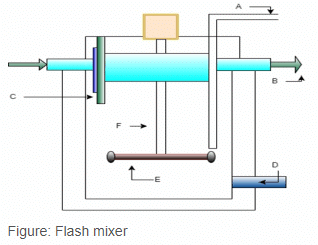# Test: Chemical Clarification

## 10 Questions MCQ Test Environmental Engineering | Test: Chemical Clarification

Description
Attempt Test: Chemical Clarification | 10 questions in 25 minutes | Mock test for Chemical Engineering preparation | Free important questions MCQ to study Environmental Engineering for Chemical Engineering Exam | Download free PDF with solutions
QUESTION: 1

###Which of the following represents the coagulant pipe?

Solution:

Explanation: ‘A’ represents the coagulant pipe which is used for discharging the coagulant into the flash mixer.

QUESTION: 2

###Which of the following represents the device which is used for sludge removal?

Solution:

Explanation: ‘D’ represents the drain valve which is used for the removal of sludge from the mixer.

QUESTION: 3

###_________ is the average of velocities at a point over a definite period of time.

Solution:

Explanation: Temporal mean velocity is the average of velocities at a point over a definite period of time. It is represented by G.

QUESTION: 4Which of the following goes to the flocculation tank?

Solution:

Explanation: ‘B’ represents the outlet of the mixer which goes to the flocculation tank.

QUESTION: 5Which of the following is the correct expression regarding temporal mean velocity?

Solution:

Explanation: Temporal mean velocity G = (P/μV) 1/2, where P is the power, μ is the dynamic viscosity and V is the volume of the tank. It is measured in per second.

QUESTION: 6Which of the following is correctly paired?

Solution:

Explanation: E represents the Impeller which is driven by the electric motor to revolve in the tank.

QUESTION: 7The speed of impeller in a flash mixer is

Solution:

Explanation: The speed of impeller in a flash mixer is between 100 to 120 rpm and the propeller type impeller is used.

QUESTION: 8‘C’ in the following figure represents the ________

Solution:

Explanation: ‘C’ represents the deflecting wall, whereas the boundary below the inlet and outlet of the tank is the flash mixer wall.

QUESTION: 9The detention period of a flash mixer is

Solution:

Explanation: The detention period of flash mixer lies between 30 seconds to 2 minutes.

QUESTION: 10Which of the following represents the impeller shaft?

Solution:

Explanation: Impeller is connected to impeller shaft which is further connected to electric motor so as to drive the impeller and mixing of coagulant in water takes place.Use Code STAYHOME200 and get INR 200 additional OFF Use Coupon Code